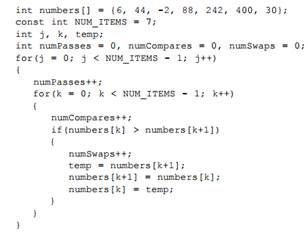All Pages

Assignment Help Basic Computer Science
Reference no: EM131397429

USING A BUBBLE SORT In this exercise, you use what you have learned about sorting data using a bubble sort. Study the following C++ code and then answer the questions that follow:1. Does this code perform an ascending sort or a descending sort? How do you know?

2. Exactly how many passes are made over the data in the array? Specify a number.

3. How many comparisons are made? Specify a number.

4. Do the variables named numPasses, numCompares, and numSwaps accurately keep track of the number of passes, compares, and swaps made in this bubble sort? Explain your answer.

Determine the thermal efficiency of this cycle

Determine the thermal efficiency of this cycle as a function of the compressor pressure ratio and the high-pressure turbine to compressor inlet temperature ratio. Compare yo

Generates all the possible n-node binary tree configurations

Implement a function that generates all the possible N-node binary tree configurations. The value N is a parameter to the function and the function should return a list of bin

What is the expected total number of tickets receive

In an arcade, you play game A 10 times and game B 20 times. Each time you play game A, you win with probability 1/3 (independently of the other times), and if you win you get

Write a program to see what actually happens on linux

Write a program to see what actually happens on Linux. If we have a variable, tid, containing a thread ID, how can a thread prevent itself from making a call, pthread_join(t

What power of h is the error proportional to

Find the values of A and B such that error is minimized. What power of h is the error proportional to? Use this formula to find d/dx sin(x) at x = pi/3. Tabulate errors for

Differences between the pp and the st that implements pp

Choose a Common Criteria protection profile and a security target of a product that implements that profile (see the Common Criteria Web site ). Identify the difference

Observer line of sight to the plane

At what rate is the angle between the observer's line of sight to the plane and the ground decreasing when the plane is horizontally 0.5 miles away from the observer? Give

Problem regarding the server in the remote machine

Create a chat client and server that can be used for communication between 2 users in different hosts. A sentence, typed by the user, will be transmitted to the server in th

Write a Review